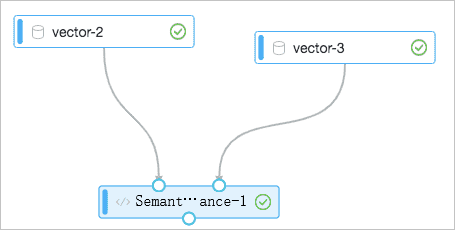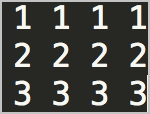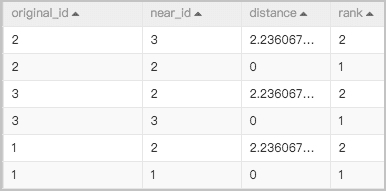The Semantic Vector Distance (Double Table) component allows you to specify two input tables. The left input port of the component is for a query table and the right input port is for a dictionary table. This output table of the component contains the top N distances between data in the query table and that in the dictionary table and the sorting of the distances.

## Considerations

When you use the Semantic Vector Distance (Double Table) component, you must take note of the following items:
• The component is used to calculate the Cartesian product distances between data in the two input tables and to sort the distances. Therefore, we recommend that you do not use more than tens of millions of samples.
• By default, a small number of resources are specified on the Tuning tab. If an out of memory (OOM) error occurs, you can increase the resources.
• If you use the Cosine distance calculation method for data of the DOUBLE type, negative numbers may exist in the output table. This is normal.

## Configure the component

The Semantic Vector Distance (Double Table) component allows you to specify two input tables. The left input port of the component is for a query table and the right input port is for a dictionary table.You can configure the component in the Machine Learning Platform (PAI) console. The following table describes the parameters for the component.
Tab Parameter Description
Fields Setting Vector Column The vector values. You must write the vector to one field. Separate all values with spaces.ID Column The primary key of each column.
Parameters Setting Distance Calculation Method Valid values: Euclidean and Cosine.
Number of Highest Similarity Scores The value of this parameter must be a positive integer.
Tuning Cores The number of CPU cores that you want to use in computing. Default value: 3. If an OOM error occurs during computing, you can increase the values of the Cores and Memory Size per Core parameters.
Memory Size per Core The memory size of each CPU core. Default value: 2046. Unit: MB. If an OOM error occurs during computing, you can increase the values of the Cores and Memory Size per Core parameters.

## Output

The following figure shows the output of the Semantic Vector Distance (Double Table) component. The output table contains the top N distances between data in the query table and that in the dictionary table and the sorting of the distances.